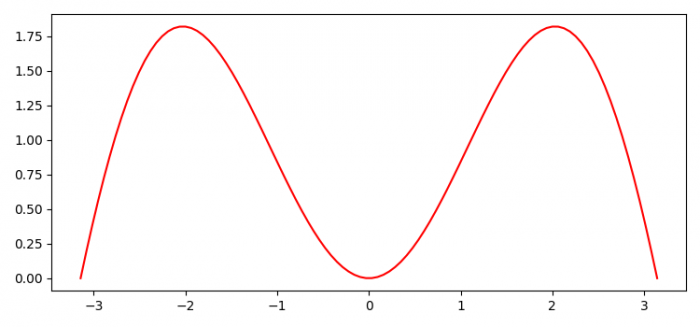# Is it possible to use pyplot without DISPLAY?

We can save the current figure in the local machine and can display it.

## Steps

• Set the figure size and adjust the padding between and around the subplots.
• Create x data points using numpy.
• Plot x and y data points using plot() method.
• Save the figure using savefig() method.

## Example

import numpy as np
import matplotlib.pyplot as plt

plt.rcParams["figure.figsize"] = [7.50, 3.50]
plt.rcParams["figure.autolayout"] = True

x = np.linspace(-np.pi, np.pi, 100)

plt.plot(x, np.sin(x) * x, c='red')

plt.savefig("myimage.png")

## Output

When we execute the code, it will save the following image as "myimage.png" in the project directory